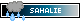(See All?) Announcements
95 Users Online

Kerberos x Maera
Print · · Subscribe · 0 Loves ·
Kerb will be making a move to attack in his next post || @Maera please post consent

Kerberos:
HP: 44
DEF: 19
ATK: 13
DMG: 11
IN: 3

Maera:
HP: 39
DEF: 19
ATK: 14
DMG: 10
IN: 4
have you checked out my player preferences?
It's good with me :)Code:
`[attacker="Kerberos" HP="44" DEF="19" ATK="13" DMG="11" IN="3"][defender="Maera" HP="39" DEF="19" ATK="14" DMG="10" IN="4"]`

Roll Results:
Kerberos rolls a d15 and gets a 6.
Kerberos's' ATK: 13
13 + 6 = 19

Maera's DEF: 19
19 = 19

The attack was a HIT!

Kerberos rolls a d6 and gets a 4.
Kerberos's DMG: 11
11 + 4 = 15

Maera's HP: 39
39 - 15 = 24

Maera's HP: 24
Kerberos's HP: 44Code:
`[attacker="Maera" HP="24" DEF="19" ATK="14" DMG="10" IN="4"][defender="Kerberos" HP="44" DEF="19" ATK="13" DMG="11" IN="3"]`

Roll Results:
Maera rolls a d15 and gets a 9.
Maera's' ATK: 14
14 + 9 = 23

Kerberos's DEF: 19
23 > 19

The attack was a HIT!

Maera rolls a d6 and gets a 6.
Maera's DMG: 10
10 + 6 = 16

Kerberos's HP: 44
44 - 16 = 28

Kerberos's HP: 28
Maera's HP: 24have you checked out my player preferences?
Code:
`[attacker="Kerberos" HP="28” DEF="19" ATK="13" DMG="11" IN="3"][defender="Maera" HP="24" DEF="19" ATK="14" DMG="10" IN="4"]`

Roll Results:
Kerberos rolls a d15 and gets a 8.
Kerberos's' ATK: 13
13 + 8 = 21

Maera's DEF: 19
21 > 19

The attack was a HIT!

Kerberos rolls a d6 and gets a 6.
Kerberos's DMG: 11
11 + 6 = 17

Maera's HP: 24
24 - 17 = 7

Maera's HP: 7
Kerberos's HP: 28”Code:
`[attacker="Maera" HP="7” DEF="19" ATK="14" DMG="10" IN="4"][defender="Kerberos" HP="28” DEF="19" ATK="13" DMG="11" IN="3"]`

Roll Results:
Maera rolls a d15 and gets a 9.
Maera's' ATK: 14
14 + 9 = 23

Kerberos's DEF: 19
23 > 19

The attack was a HIT!

Maera rolls a d6 and gets a 2.
Maera's DMG: 10
10 + 2 = 12

Kerberos's HP: 28”
28” - 12 = 16

Kerberos's HP: 16
Maera's HP: 7”